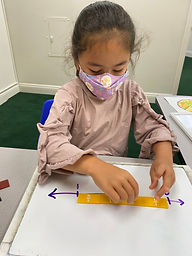## Ms. Brianne

### Target 1​

###### Lesson Type:

Continuation

Number Operation

:

Fractional Numbers

Understand that a fraction is a quantity formed when a whole is partitioned into equal parts.

###### 1:

Compose a written fraction to describe a visual or a visual to describe a written fraction.

###### 2:

Understand that fractions can be represented on a number line.

###### 3:

Explain that a fraction number line illustrates the value of fractions.

###### 4:

Determine the placement of a fraction on a number line.

4th

###### Vocabulary:

Fraction, Number Line, Numerator, Denominator

Activities:

• Students completed their fraction pizza, stating the fraction for each topping on their pizza.
• Students were shown a number line with a frog at a given location and they identified the fraction at that location. This helped them realized that fractions are less than one whole and are in between 0 and 1.
• Students used fraction tiles to help them create different number lines with fractional pieces.
• Students colored in fraction models and wrote the matching fractions on a number line from 0 to 1.### Home Exploration

###### Guiding Questions:## Absent Students:

### Target 2

:

###### 1:

Solve simple tangram puzzles by recognizing which spaces in the puzzle the given seven shapes can and cannot fit.

###### 2:

Solve pentomino puzzles by recognizing which spaces in the puzzle the given 12 pieces can and cannot fit.

4th

###### Vocabulary:

Tangrams, Pentominoes

Activities:

• Students worked with tangrams to solve tangram puzzles.
• Students fit pentominoes together so that there were no spaces in between the pieces.
• Students used 12 pentominoes to try and completely fill in a rectangular grid.### Home Exploration

###### Guiding Questions:### Target 3

:

###### Vocabulary:

Activities:### Home Exploration